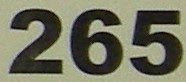## Tuesday, March 9, 2010

### 265

265 = 5 x 53.

2, 6, and 5 are the sixth, seventh, and eighth decimal digits of pi.

265 has two representations as a sum of two squares: 265 = 32 + 162 = 112 + 122.

265 is the hypotenuse of two primitive Pythagorean triples: 2652 = 232 + 2642 = 962 + 2472.Greek mathematician Archimedes, who is studying in Alexandria in the year 265 BC, devised a screw for raising water

Source: Wikipedia